﻿ 基于分类差异与信息熵对抗的无监督域适应算法
«上一篇文章快速检索 高级检索

 智能系统学报2021, Vol. 16Issue (6): 999-1006  DOI: 10.11992/tis.2020100200

### 引用本文LI Qingyong, HE Jun, ZHANG Chunxiao. Unsupervised domain adaptation algorithm based on classification discrepancy and information entropy[J]. CAAI Transactions on Intelligent Systems, 2021, 16(6): 999-1006. DOI: 10.11992/tis.202010020.### 文章历史

1. 南京信息工程大学 电子与信息工程学院，江苏 南京 210044;
2. 南京信息工程大学 人工智能学院，江苏 南京 210044

Unsupervised domain adaptation algorithm based on classification discrepancy and information entropy
LI Qingyong 1, HE Jun 1,2, ZHANG Chunxiao 1
1. School of Electronics and Information Engineering, Nanjing University of Information Science and Technology, Nanjing 210044, China;
2. School of Artificial Intelligence, Nanjing University of Information Science and Technology, Nanjing 210044, China
Abstract: The adversarial training method has become the mainstream of the domain adaptation algorithm. The feature distributions of the source and target domains are aligned by a domain classifier to reduce the feature distribution discrepancy among different domains. However, existing domain adaptation methods only reduce the distance between different domain data without considering the relationship between the data distribution of the target domain and decision boundaries, thus decreasing the intradomain distinguishability of different categories in the target domain. Considering the shortcomings of the existing methods, an unsupervised domain adaptation algorithm based on classification discrepancy and information entropy confrontation (ACDIE) is proposed in this study. The algorithm uses the discrepancy and the domain aligning discrepancy between two classifiers and minimizes the information entropy to reduce uncertainty. Consequently, the proposed method makes the target domain feature far away from the decision boundaries and improves the distinguishability of different categories. The experimental results of the digital identification and Office-31 datasets show that the ACDIE algorithm can learn better feature representation. Moreover, the accuracy of the domain adaptation classification is considerably improved.
Key words: domain adaptation    confrontation training    neural network    unsupervised learning    transfer learning    classification discrepancy    information entropy    decision boundary

1 分类差异和信息熵对抗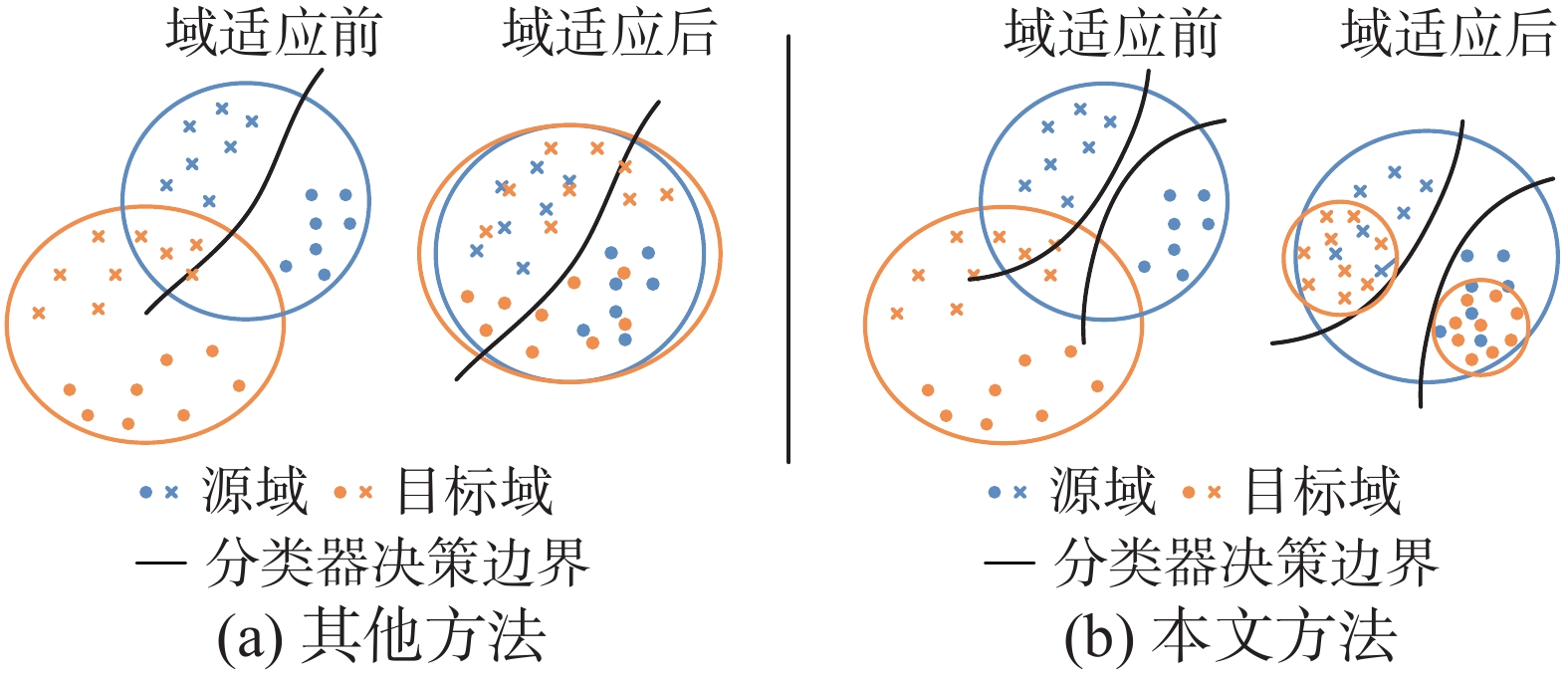Download: 图 1 不同方法特征分布对比 Fig. 1 Comparison of the feature distribution of different methods
1.1 信息熵对抗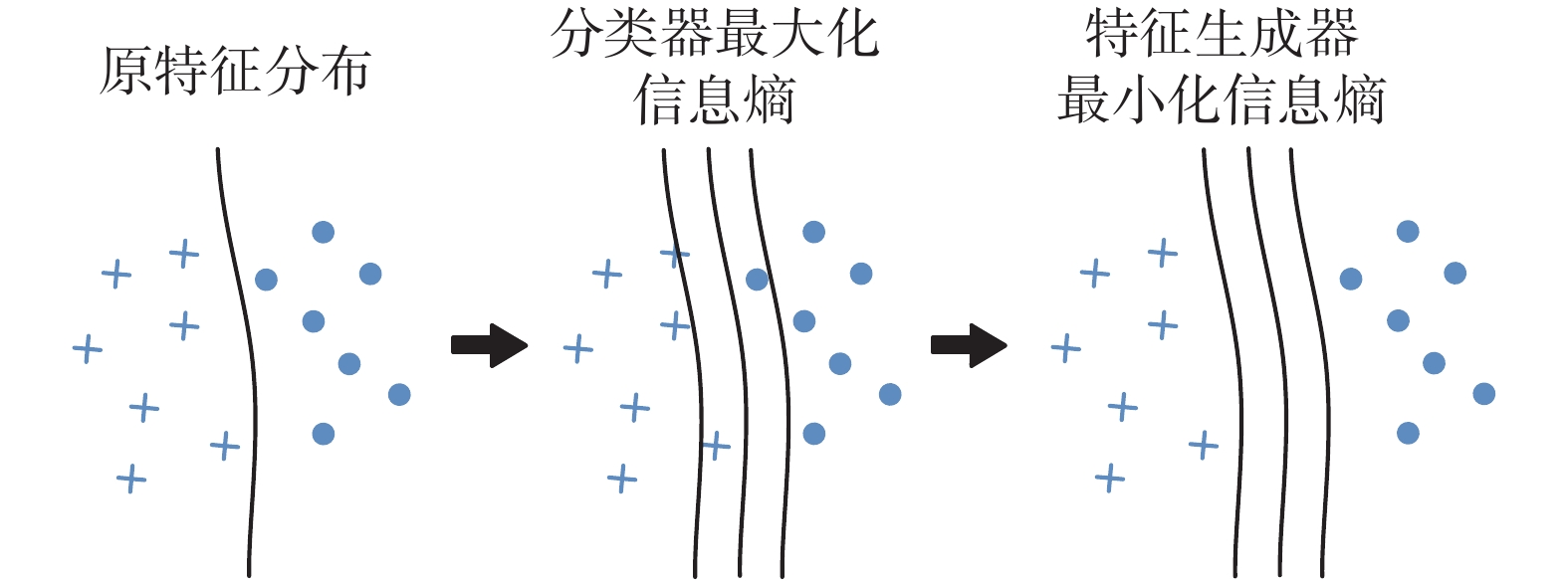Download: 图 2 信息熵对抗过程 Fig. 2 Information entropy confrontation process
1.2 算法分析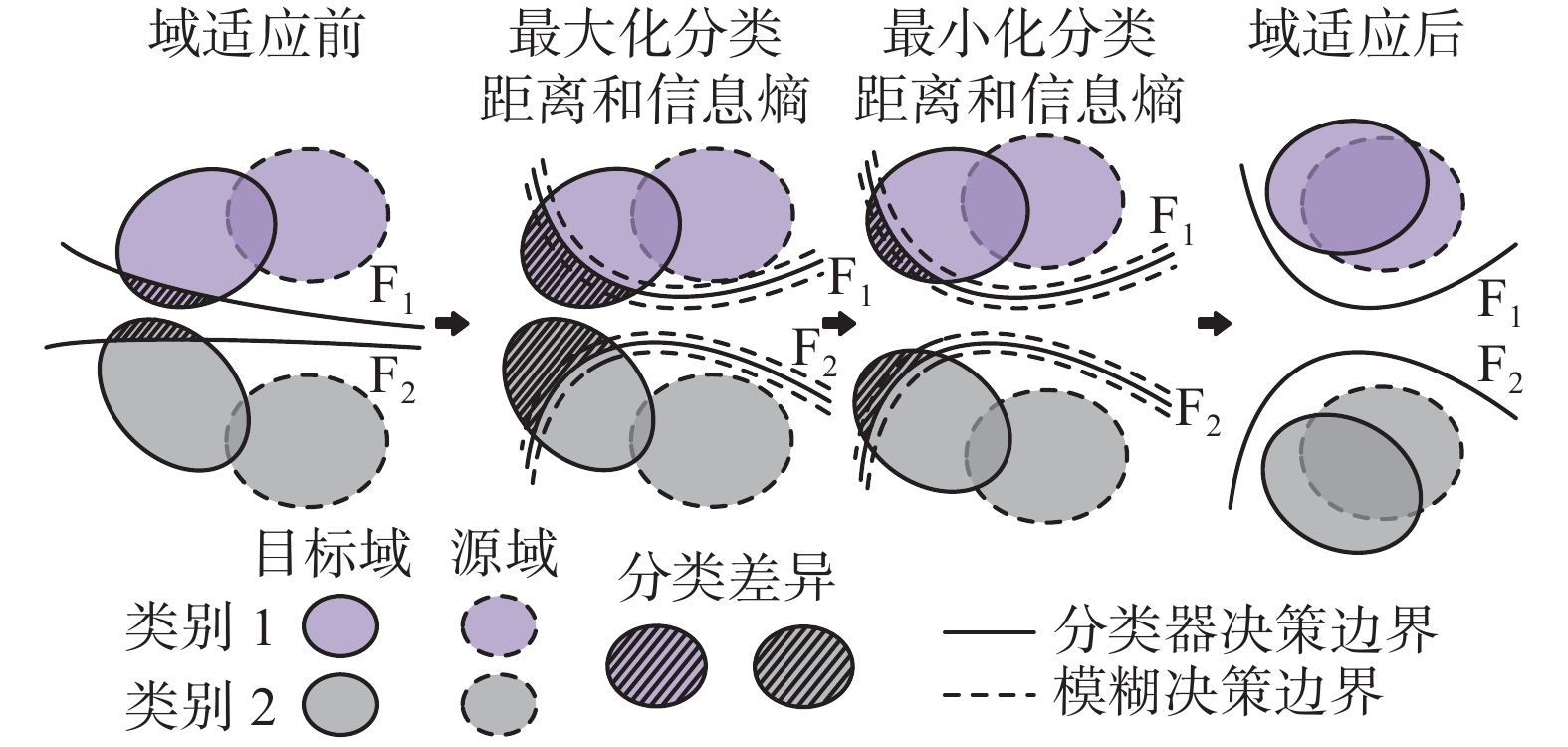Download: 图 3 本文算法特征分布对齐过程 Fig. 3 Alignment process of the feature distribution is presented in this paper

 $\forall h \in H,{R_T}(h) \leqslant {R_S}(h) + \frac{1}{2}{d_{{\mathcal{H}}}}(S,T) + \lambda$ (1)
 ${d_{{\mathcal{H}}}}(S,T) = 2\mathop {\sup }\limits_{(h,h') \in {H^2}} \left| {\mathop E\limits_{x \sim S} I[h(x) \ne h'(x)] - \mathop E\limits_{x \sim T} I[h(x) \ne h'(x)]} \right|$ (2)
 $\lambda = \min [{R_S}(h) + {R_T}(h)]$ (3)

 ${d_{{\mathcal{H}}}}(S,T) = \mathop {\sup }\limits_{(h,h') \in {H^2}} \mathop E\limits_{x \sim T} I[h(x) \ne h'(x)]$ (4)

 $\mathop {\sup }\limits_{{{F_1,F_2}}} \mathop E\limits_{x \sim T} I[{{F_1}} \circ G(x) \ne {{F_2}} \circ G(x)]$ (5)

 $\mathop {\min }\limits_G \mathop {\max }\limits_{{{F_1,F_2}}} \mathop E\limits_{x \sim T} I[{{F_1}} \circ G(x) \ne {{F_2}} \circ G(x)]$ (6)

1.3 Softmax交叉熵损失

 ${L_{{\rm{cl}}}}({X_s},{Y_s}) = - \frac{1}{K}\sum\limits_{i = 1}^K {I(i = y_s^{(i)})\log {p_s}(x_s^{(i)})}$ (7)

1.4 分类差异损失

 ${L_d}({X_t}) = d({p_1}(y|{x_t}),{p_2}(y|{x_t})){\rm{ = }}\frac{1}{K}\sum\limits_{k = 1}^K {\left| {{p_{1k}} - {p_{2k}}} \right|}$ (8)

1.5 信息熵损失

 ${L_{{\rm{ent}}}}({X_t}) = H({X_t}) = \frac{1}{K}\sum\limits_{i = 1}^K { - F(G(x_t^{(i)}))\log F(G(x_t^{(i)}))}$ (9)

1.6 算法流程

${L_{{\rm{cl1}}}}$ ${L_{{\rm{cl2}}}}$ 分别表示分类器 $\rm {F_{{1}}}$ $\rm{F_{{2}}}$ 的Softmax交叉熵损失， ${L_{{\rm{ent1}}}}$ ${L_{{\rm{ent2}}}}$ 分别表示分类器 $\rm{F_{{1}}}$ $\rm{F_{{2}}}$ 的信息熵损失。输入源域数据集 ${D_s} = \{ {X_s},{Y_s}\}$ ，目标域数据集 ${D_t} = \{ {X_t}\}$ ，批次大小为m，特征提取器训练次数为n。ACDIE模型训练的整体算法流程为：

1)从 ${D_s}$ 中采样m个有标注数据 $\{ {x_{si}},{y_{si}}\} _{i = 1}^m$ ，记为 ${\rm{\{ }}{X_{sm}}{\rm{,}}{Y_{sm}}{\rm{\} }}$ ;从 ${D_t}$ 中采样m个无标注数据 $\{ {x_{ti}}\} _{i = 1}^m$ ，记为 ${\rm{\{ }}{X_{tm}}{\rm{\} }}$

2)通过有标注数据进行监督训练；

3)计算损失函数 ${L_1} = {L_{{\rm{cl1}}}} + {L_{{\rm{cl2}}}}$

4)反向传播梯度信号，更新 ${\rm{G}}$ $\rm{F_{{1}}}$ $\rm{F_{{2}}}$ 中的参数；

5)通过无标注数据进行域适应训练；

6)计算损失函数 ${L_{\rm{2}}}= {L_{{\rm{cl1}}}}+ {L_{{\rm{cl2}}}} - {L_d}({X_{tm}}) - {L_{{\rm{ent1}}}} ({X_{tm}}) -$ ${L_{{\rm{ent2}}}}({X_{tm}})$

7)计算损失函数 ${L_{\rm{3}}}={L_d}({X_{tm}}) + {L_{{\rm{ent1}}}}({X_{tm}}) + {L_{{\rm{ent2}}}}({X_{tm}})$

8)反向传播梯度信号，更新 ${\rm{G}}$ 中的参数；

9)重复训练步骤7）~8）n次。

2 训练步骤

1)模型预训练

 $\mathop {\min }\limits_{G,{F_1},{F_2}} {L_{{\rm{cl}}}}({X_s},{Y_s})$ (10)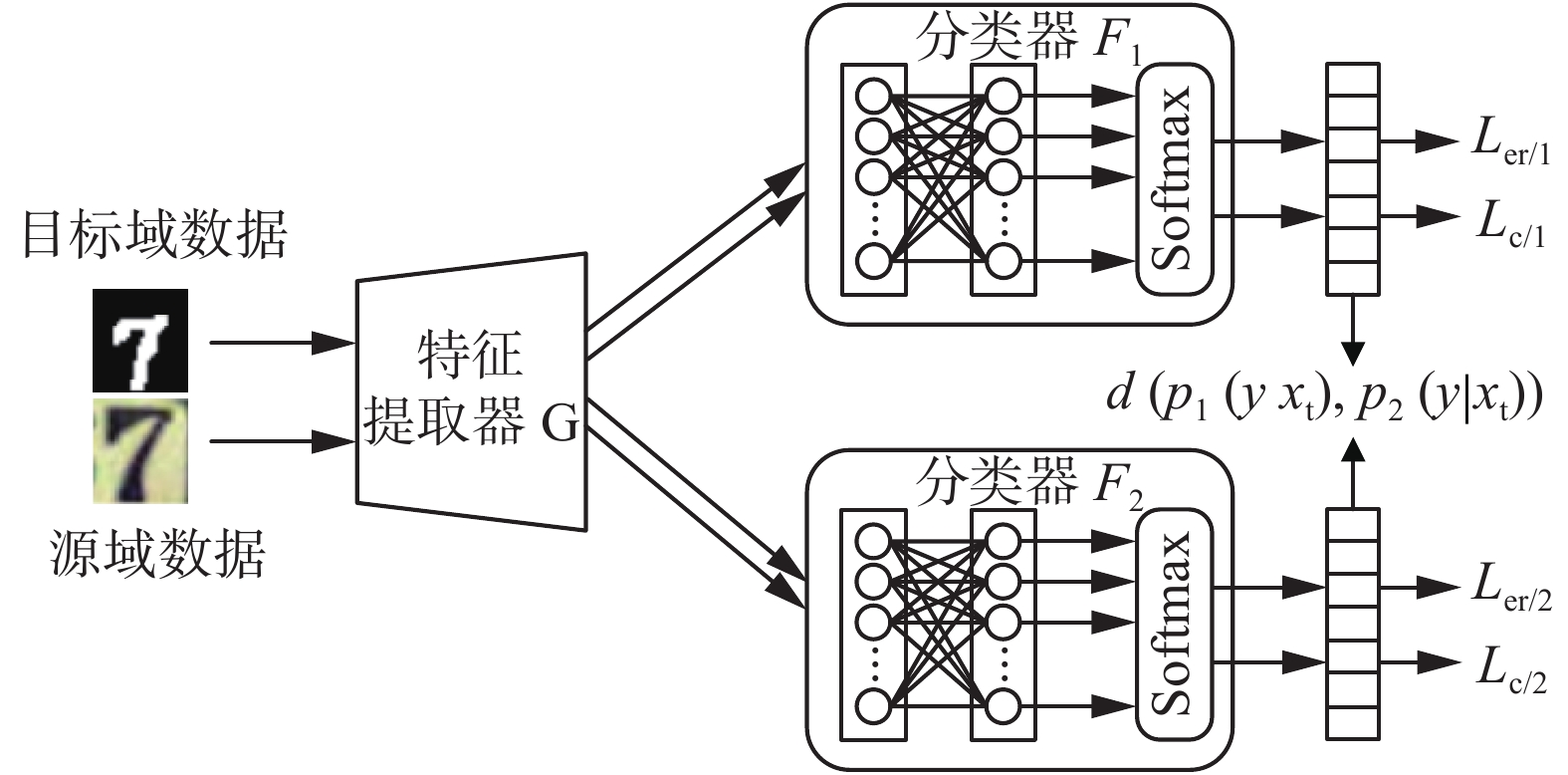Download: 图 4 ACDIE模型流程 Fig. 4 ACDIE model flow

2)训练分类器

 $\mathop {\min }\limits_{{F_1},{F_2}} {L_{{\rm{cl}}}}({X_s},{Y_s}) - {L_d}({X_t}) - {L_{{\rm{ent1}}}}({X_t}) - {L_{{\rm{ent2}}}}({X_t})$ (11)

3)训练特征生成器

 $\mathop {\min }\limits_G {L_d}({X_t}) + {L_{{\rm{ent1}}}}({X_t}) + {L_{{\rm{ent2}}}}({X_t})$ (12)

3 实验设计与结果分析

3.1 数字标识域适应实验 3.1.1 数据集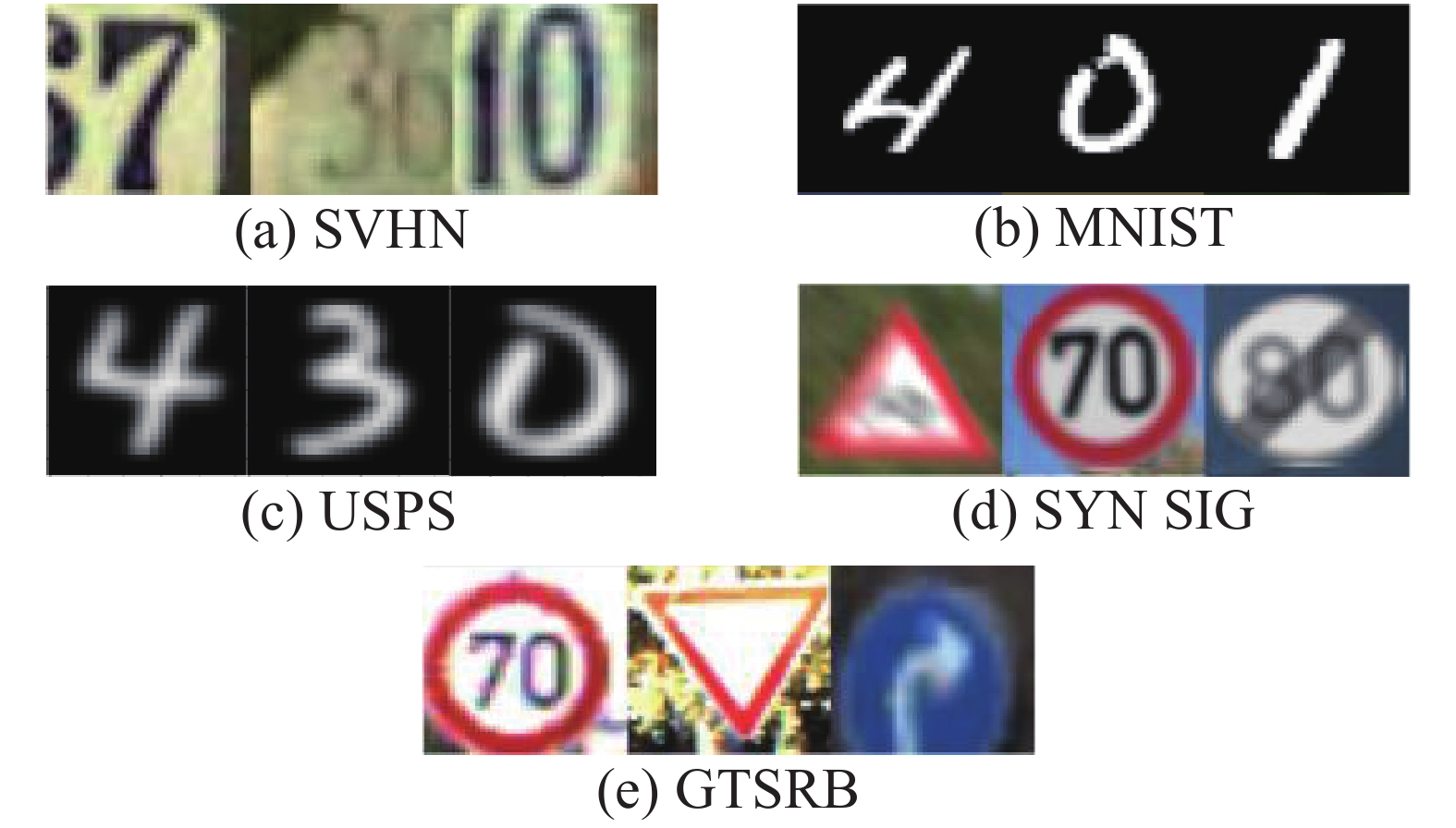Download: 图 5 数字标识数据集示例 Fig. 5 Digital ID dataset example

3.1.2 实验超参数

3.1.3 对比实验结果表 1 数字标识数据集域适应准确率对比 Tab.1 Comparison of the domain adaptation accuracies of digital ID datasets
3.2 实物域适应实验 3.2.1 Office-31数据集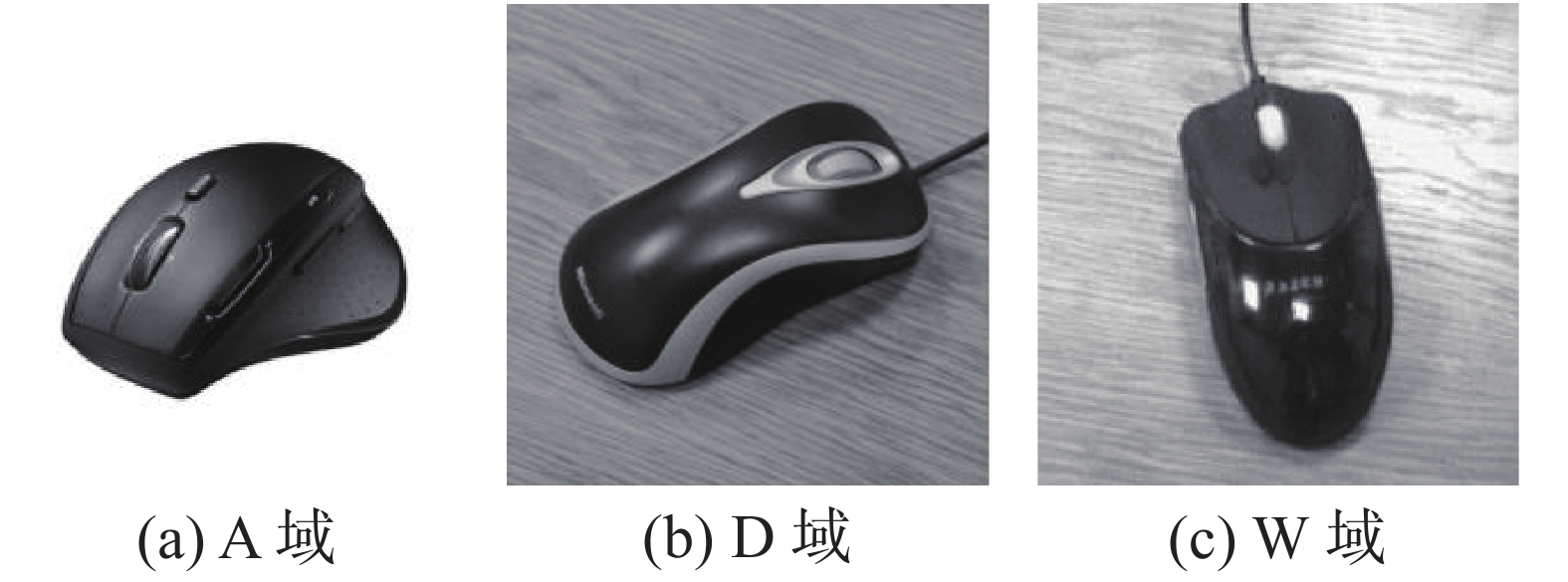Download: 图 6 Office-31数据集示例 Fig. 6 Office-31 dataset example
3.2.2 实验超参数

3.2.3 对比实验结果表 2 Office-31数据集域适应准确率对比 Tab.2 Comparison of the domain adaptation accuracies of Office-31 dataset

3.3 t-SNE图可视化实验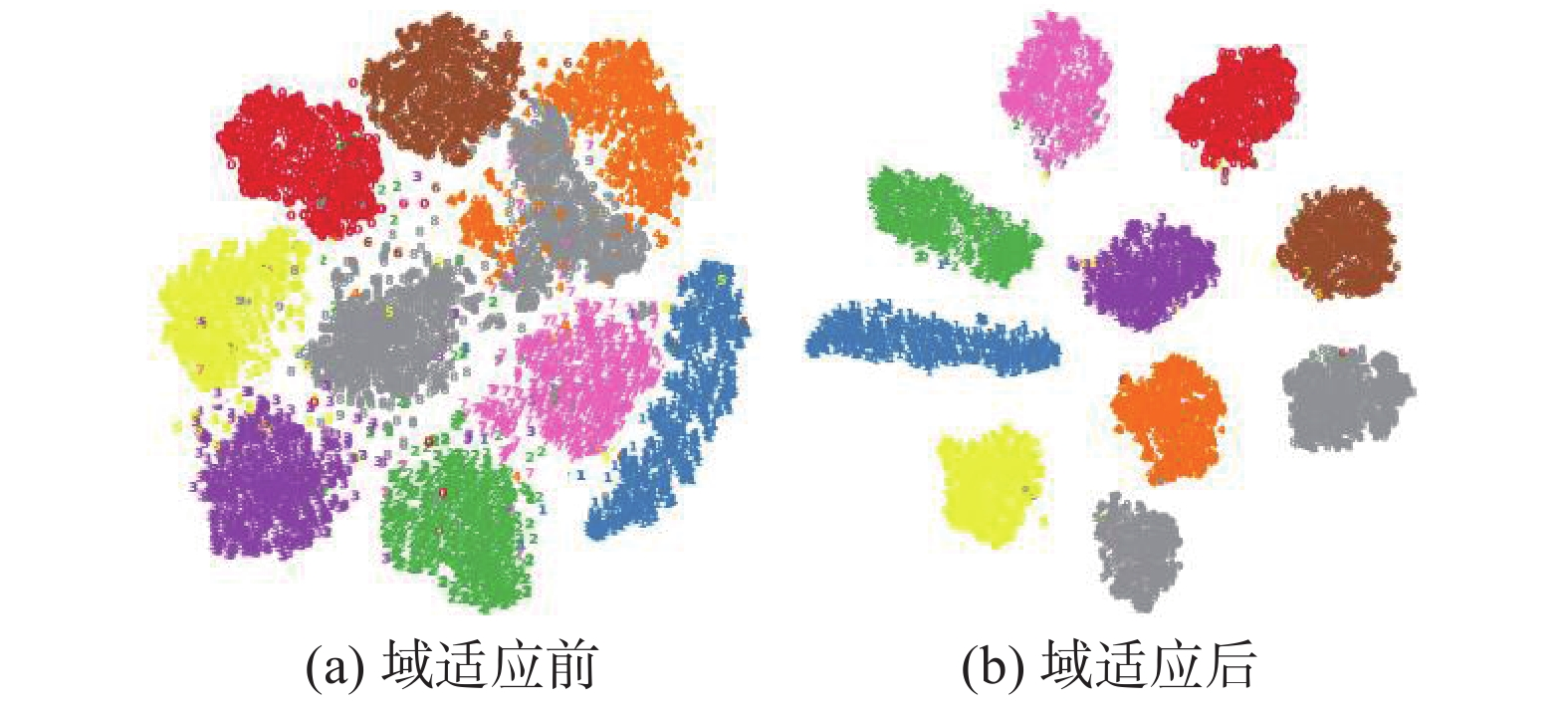Download: 图 7 SVHN $\to$ MNIST的t-SNE图 Fig. 7 t-SNE diagram of SVHN $\to$ MNIST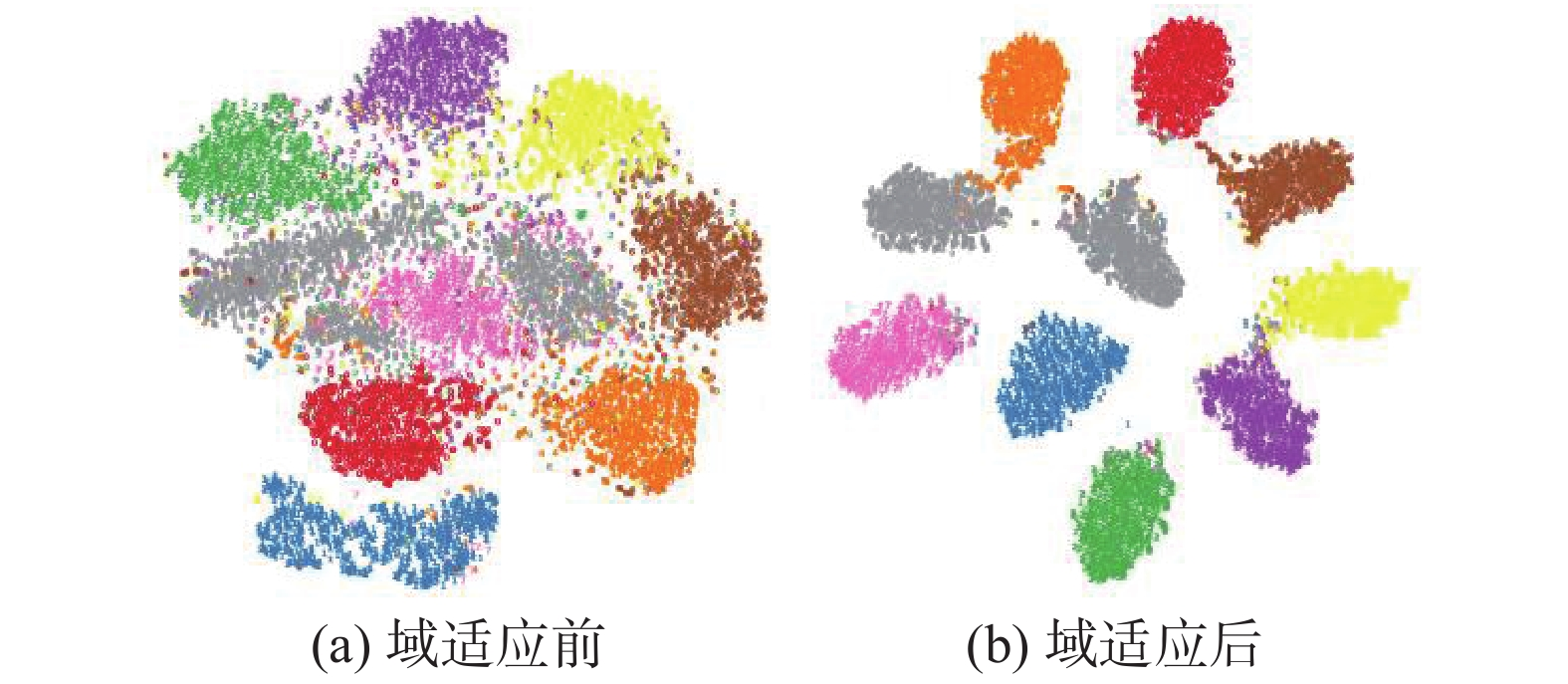Download: 图 8 USPS $\to$ MNIST的t-SNE图 Fig. 8 t-SNE diagram of USPS $\to$ MNIST
3.4 信息熵损失对比实验表 3 信息熵损失对比实验 Tab.3 Comparative experiment of information entropy loss
4 结束语

  WANG Xiaolong, GUPTA A. Unsupervised learning of visual representations using videos[C]//Proceedings of the IEEE International Conference on Computer Vision. Santiago, Chile: IEEE, 2015: 2794−2802. (0)  MAHJOURIAN R, WICKE M, ANGELOVA A. Unsupervised learning of depth and ego-motion from monocular video using 3D geometric constraints[C]//Proceedings of 2018 IEEE/CVF Conference on Computer Vision and Pattern Recognition. Salt Lake City, USA: IEEE, 2018: 5667−5675. (0)  刘建伟, 孙正康, 罗雄麟. 域自适应学习研究进展[J]. 自动化学报, 2014, 40(8): 1576-1600. LIU Jianwei, SUN Zhengkang, LUO Xionglin. Review and research development on domain adaptation learning[J]. Acta automatica sinica, 2014, 40(8): 1576-1600. (0)  PAN S J, YANG Qiang. A survey on transfer learning[J]. IEEE transactions on knowledge and data engineering, 2010, 22(10): 1345-1359. DOI:10.1109/TKDE.2009.191 (0)  ROZANTSEV A, SALZMANN M, FUA P. Beyond sharing weights for deep domain adaptation[J]. IEEE transactions on pattern analysis and machine intelligence, 2019, 41(4): 801-814. DOI:10.1109/TPAMI.2018.2814042 (0)  GHIFARY M, KLEIJN W B, ZHANG Mengjie, et al. Deep reconstruction-classification networks for unsupervised domain adaptation[C]//Proceedings of the 14th European Conference on Computer Vision. Amsterdam, The Netherlands: Springer, 2016: 597−613. (0)  SENER O, SONG H O, SAXENA A, et al. Learning transferrable representations for unsupervised domain adaptation[C]//Proceedings of the 30th International Conference on Neural Information Processing Systems. Barcelona, Spain: Curran Associates Inc., 2016: 2110−2118. (0)  SUN Baochen, FENG Jiashi, SAENKO K. Return of frustratingly easy domain adaptation[C]//Proceedings of the Thirtieth AAAI Conference on Artificial Intelligence. Phoenix, Arizona: AAAI Press, 2016: 2058−2065. (0)  GRETTON A, BORGWARDT K M, RASCH M J, et al. A kernel two-sample test[J]. The journal of machine learning research, 2012, 13: 723-773. (0)  TZENG E, HOFFMAN J, ZHANG Ning, et al. Deep domain confusion: maximizing for domain invariance[J]. Computer science, 2014. (0)  LONG Mingsheng, CAO Yue, WANG Jianmin, et al. Learning transferable features with deep adaptation networks[C]//Proceedings of the 32nd International Conference on Machine Learning. Lille, France: JMLR, 2015: 97−105. (0)  LONG Mingsheng, ZHU Han, WANG Jianmin, et al. Unsupervised domain adaptation with residual transfer networks[C]//Proceedings of the 30th International Conference on Neural Information Processing Systems. Barcelona, Spain: Curran Associates Inc., 2016: 136−144. (0)  GOODFELLOW I J, POUGET-ABADIE J, MIRZA M, et al. Generative adversarial nets[C]//Proceedings of the 27th International Conference on Neural Information Processing Systems. Montreal, Canada: MIT Press, 2014: 2672−2680. (0)  GANIN Y, USTINOVA E, AJAKAN H, et al. Domain-adversarial training of neural networks[J]. The journal of machine learning research, 2016, 17(1): 2096-2030. (0)  王格格, 郭涛, 余游, 等. 基于生成对抗网络的无监督域适应分类模型[J]. 电子学报, 2020, 48(6): 1190-1197. WANG Gege, GUO Tao, YU You, et al. Unsupervised domain adaptation classification model based on generative adversarial network[J]. Acta electronica sinica, 2020, 48(6): 1190-1197. DOI:10.3969/j.issn.0372-2112.2020.06.021 (0)  SANKARANARAYANAN S, BALAJI Y, CASTILLO C D, et al. Generate to adapt: aligning domains using generative adversarial networks[C]//Proceedings of 2018 IEEE/CVF Conference on Computer Vision and Pattern Recognition. Salt Lake City, USA: IEEE, 2018: 8503−8512. (0)  SAITO K, WATANABE K, USHIKU Y, et al. Maximum classifier discrepancy for unsupervised domain adaptation[C]//Proceedings of 2018 IEEE/CVF Conference on Computer Vision and Pattern Recognition. Salt Lake City, USA: IEEE, 2018: 3723−3732. (0)  BEN-DAVID S, BLITZER J, CRAMMER K, et al. A theory of learning from different domains[J]. Machine learning, 2010, 79(1/2): 151-175. (0)  LECUN Y, BOTTOU L, BENGIO Y, et al. Gradient-based learning applied to document recognition[J]. Proceedings of the IEEE, 1998, 86(11): 2278-2324. DOI:10.1109/5.726791 (0)  HULL J J. A database for handwritten text recognition research[J]. IEEE transactions on pattern analysis and machine intelligence, 1994, 16(5): 550-554. DOI:10.1109/34.291440 (0)  NETZER Y, WANG T, COATES A, et al. Reading digits in natural images with unsupervised feature learning[C]//Proceedings of the NIPS Workshop on Deep Learning and Unsupervised Feature Learning. Granada, Spain, 2011: 5−16. (0)  MOISEEV B, KONEV A, CHIGORIN A, et al. Evaluation of traffic sign recognition methods trained on synthetically generated data[C]//Proceedings of the 15th International Conference on Advanced Concepts for Intelligent Vision Systems. Poznań, Poland: Springer, 2013: 576−583. (0)  STALLKAMP J, SCHLIPSING M, SALMEN J, et al. The German traffic sign recognition benchmark: a multi-class classification competition[C]//Proceedings of 2011 International Joint Conference on Neural Networks. San Jose, USA: IEEE, 2011: 1453−1460. (0)  BOUSMALIS K, TRIGEORGIS G, SILBERMAN N, et al. Domain separation networks[C]//Proceedings of the 30th International Conference on Neural Information Processing Systems. Barcelona, Spain: Curran Associates Inc., 2016: 343−351. (0)  TZENG E, HOFFMAN J, SAENKO K, et al. Adversarial discriminative domain adaptation[C]//Proceedings of 2017 IEEE Conference on Computer Vision and Pattern Recognition. Honolulu, USA: IEEE, 2017: 7167−7176. (0)  LIU Mingyu, TUZEL O. Coupled generative adversarial networks[C]//Proceedings of the 30th International Conference on Neural Information Processing Systems. Barcelona, Spain: Curran Associates Inc., 2016: 469−477. (0)  LONG Mingsheng, CAO Zhangjie, WANG Jianmin, et al. Conditional adversarial domain adaptation[C]//Proceedings of the 32nd International Conference on Neural Information Processing Systems. Montréal, Canada: Curran Associates Inc., 2018: 1647−1657. (0)  VAN DER MAATEN L, HINTON G. Visualizing data using t-SNE[J]. Journal of machine learning research, 2008, 9(2605): 2579-2605. (0)